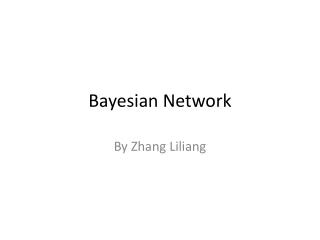DownloadDownload PresentationBayesian Network

# Bayesian Network

Télécharger la présentation## Bayesian Network

- - - - - - - - - - - - - - - - - - - - - - - - - - - E N D - - - - - - - - - - - - - - - - - - - - - - - - - - -
##### Presentation Transcript

1. Bayesian Network By Zhang Liliang

2. Key Point Today • Intro to Bayesian Network • Usage of Bayesian Network • Reasoning BN: D-separation

3. Bayesian Network Definition Bayes networks defines Joint Distribution in term of a graph over a collection of random variable. Difficulty {easy, hard} Intelligence {low, high} Grade {A, B, C} SAT {low_mark, high_mark} Letter {No, Yes} P(D, I, G, S, L)= ?

4. Joint Distribution of BN General Form: P(D, I, G, S, L) = P(L|D, I, G, S) *P(S|D, I, G) *P(G|D, I) *P(I|D) *P(D) D I G S L Can the formula be simplify?

5. Conditional Independence For (sets of) random variables X,Y,Z X is conditional independence of Y given Z,Denotes as P (X ⊥ Y | Z), if: • – P(X, Y|Z) = P(X|Z) P(Y|Z) • – P(X|Y,Z) = P(X|Z) • – P(Y|X,Z) = P(Y|Z)

6. Joint Distribution of BN General Form: P(D, I, G, S, L) = P(L|D, I, G, S) *P(S|D, I, G) *P(G|D, I) *P(I|D) *P(D) D I G S L Parameters: 2*2*3*2*2-1=47 In BN, it can be simplify as: P(D, I, G, S, L) = P(D) * P(I) * P(G|D, I) * P(S|I) * P(L|G) Parameters: 1+1+8+2+3 = 15

7. Bayesian Network Definition(2) A Bayesian network is a directed acyclic graph(DAG) and a set of conditional probability distribution(CPD). P(D, I, G, S, L) = P(D) * P(I) * P(G|D, I) * P(S|I) * P(L|G)

8. Usages of BN: Reasoning 3 kinds of reasoning: • Causal Reasoning • Evidential Reasoning • Intercausal Reasoning D I G S L

9. Causal Reasoning

10. Evidential Reasoning

11. Intercausal Reasoning

12. How to reasoning? • For certain cases, tractable - Full observed set of variable - just one variable unobserved • In general, intractable…(NP-complete) How to deal with the problem? An intuitive solution: D-separation

13. Conditional Independence: Revisited For (sets of) random variables X,Y,Z X is conditional independence of Y given Z,Denotes as P (X ⊥ Y | Z), if: • – P(X, Y|Z) = P(X|Z) P(Y|Z) • – P(X|Y,Z) = P(X|Z) • – P(Y|X,Z) = P(X|Z) D I G S L A method may simplify the calculation when reasoning : to find out more variables which satisfied with conditional independence. Given an observation of G, is L is conditional independence of D? Given an observation of I, is G conditional independence of S ? Given an observation of G, is D conditional independence of I ?

15. Head to Tail (D⊥L ) ? (D⊥L| G) ? No Yes D I G S L

16. Tail to Tail (G⊥S) ? (G⊥S| I) ? No Yes D I G S L

17. Head to Head (D⊥I)? (D⊥I| G) ? Yes No D I G S L

18. X and Y are conditionally independent given Z, if and only if X and Y are D-separated by Z Suppose we have three sets of random variables: X, Y and Z X and Y are D-separated by Z (and therefore conditionally independence, given Z) iff every path from any variable in X to any variable in Y is blocked A path from variable A to variable B is blocked if it includes a node such that either 1.arrows on the path meet either head-to-tail or tail-to-tail at the node and this node is in Z 2.the arrows meet head-to-head at the node, and neither the node, nor any of its descendants, is in Z

19. Summary • Bayesian Network = Directed Acyclic Graph + Conditional Probability Distribution • Joint Distribution: • Three type of Reasoning BN: causal, evidential, intercausal • Conditional Independence & D-separation

20. Reference • Machine Learning, CMU • Probabilistic Graphical Models, Stanford, on Coursera. • SamIam: a comprehensive tool, UCLA

21. Thanks